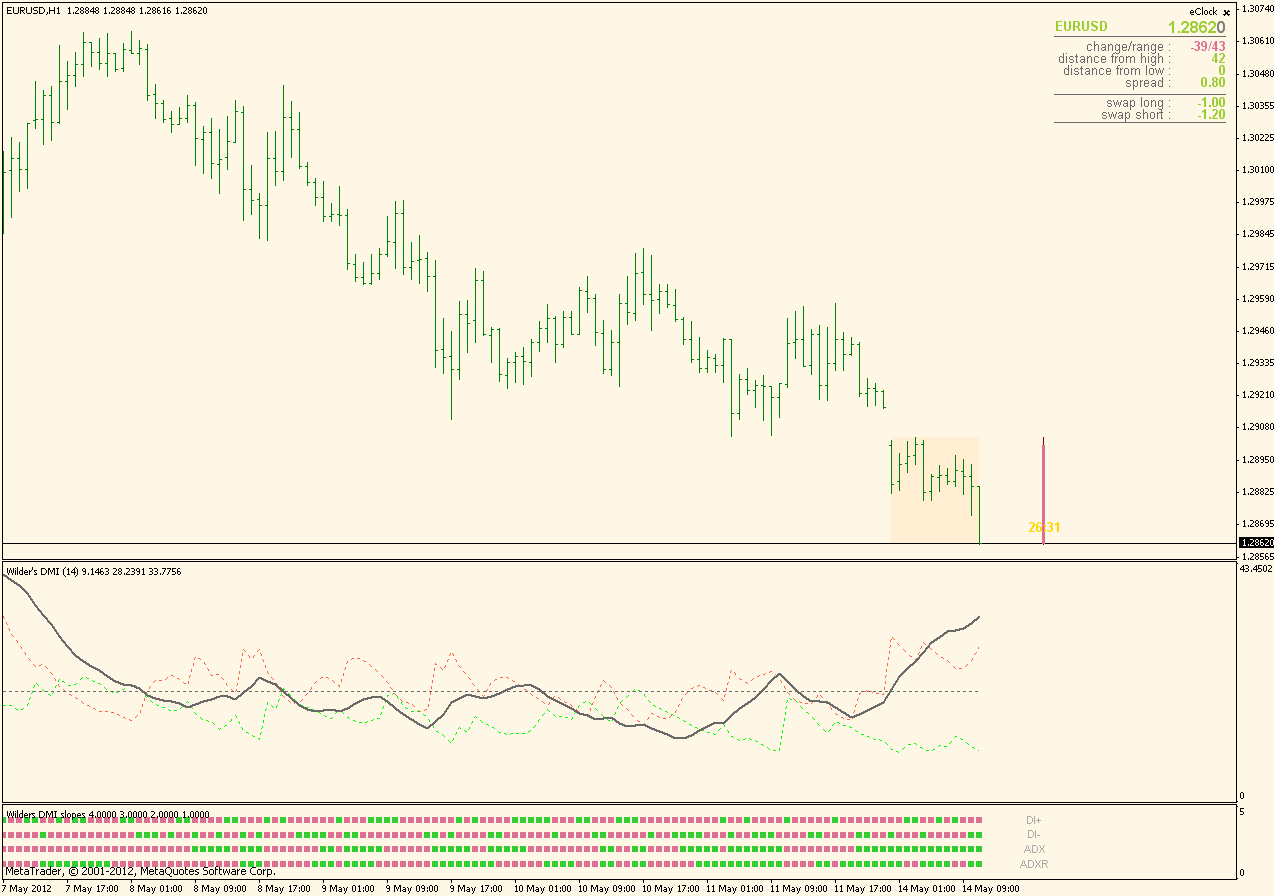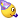# Requests & Ideas - page 364193

Do you think its possible ?

Best regards

rosalieone I am not sure it can be done. Will check it17

Can This Be Done for the new version of Mt4? Cant Test it

(Wilders Slope)

winner246

What you are asking in this post is impossible to combine with slopes and with all the features you are asking for (not enough drawing buffers). To have that you have to simply use the regular Wilder's DMI.

Anyway, this is the Wilders DMI that shows slopes for DI+,Di-,ADX and ADXR as per your original request. Labels are added to any of the slope showing rows so it is visible which row is showing a slope for which valueregards176

Mladen, sorry if i make you repeating the same thing, is it possible combining advanced forecater and envelopes separate?

Best Regards163384

tomitom06:
Mladen, sorry if i make you repeating the same thing, is it possible combining advanced forecater and envelopes separate? Best Regards

tomitom06176

advanced forecater 2.04 mtf (one of your artworks). If not, i want to see something to combine with envelopes separate... It gives very good signals.

Best Regards163384

tomitom06:

advanced forecater 2.04 mtf (one of your artworks). If not, i want to see something to combine with envelopes separate... It gives very good signals.

Best Regards

OK, will check it176OK, will check it17

Mrtools or Mladen I found this New indicator review for visual chart. I didnt find anything similar coded in mt4.

Both ( 2 Periods of Macd Momentum and Histo Versions ) are very interesting.

Visual Chart: El indicador MACD Momentum Ratio

Here you have the code:

Dim MomPeriod As Integer '5

Dim MACD1_N1 As Integer '2

Dim MACD1_N2 As Double '1.5

Dim MACD2_N1 As Integer '6

Dim MACD2_N2 As Double '1.25

Dim N As Integer '100

'Parameters !!

Dim momdata As DataIdentifier

Dim macd1data As DataIdentifier

Dim macd2data As DataIdentifier

Dim dif() As Double

Dim dif2() As Double

Dim lastbar As Long

Option Explicit

Public APP As OscUserApp

Implements Indicator

Public Sub Indicator_OnInitCalculate()

With APP

momdata = .GII(Momentum, Data, MomPeriod, PriceClose, 100)

macd1data = .GII(MACD, momdata, MACD1_N1 * MomPeriod, (MACD1_N1 * MomPeriod) * MACD1_N2, 1, PriceClose, 0)

macd2data = .GII(MACD, momdata, MACD2_N1 * MomPeriod, (MACD2_N1 * MomPeriod) * MACD2_N2, 1, PriceClose, 0)

ReDim dif(N - 1)

lastbar = -1

.SetLineName 1, "macdmom_r"

End With

End Sub

Public Sub Indicator_OnCalculateBar(ByVal Bar As Long)

With APP

Dim curmacd2 As Double

Dim curmacd1 As Double

curmacd2 = .GIV(macd2data)

curmacd1 = .GIV(macd1data)

If (curmacd2 NullValue) Then

If lastbar Bar Then

dif2 = dif

Else

dif = dif2

End If

Dim i As Integer

For i = N - 1 To 1 Step -1

dif(i) = dif(i - 1)

Next i

Dim ippos As IndicatorPosition

Dim signal As Integer

If (curmacd1 > curmacd2) Then

ippos = ipBull

signal = 1

Else

ippos = ipBear

signal = -1

End If

dif(0) = Math.Abs((curmacd1 - curmacd2))

Dim ratio As Double

Dim maxdif As Double

maxdif = GetMaxDif()

If maxdif 0 Then

ratio = dif(0) / maxdif

.SetIndicatorValue signal * ratio, Position:=ippos

.SetBarRepresentation 0, 1, irVolume

End If

End If

lastbar = Bar

End With

End Sub

Public Sub Indicator_OnSetParameters(ParamArray ParamList() As Variant)

'¡¡ Parameters initialization

'Parameters initialization !!

End Sub

Public Sub Indicator_OnCalculateRange(ByVal StartBar As Long, ByVal FinalBar As Long)

Dim i As Long

i = APP.StartBar

If StartBar > i Then

i = StartBar

End If

While Not APP.ShouldTerminate And i <= FinalBar

APP.CurrentBar = i

Indicator_OnCalculateBar i

i = i + 1

Wend

End Sub

Private Sub OscUserAppInstance_OnConnection(ByVal Application As OscUserApp, ByVal MTDllInst As Object, Custom() As Variant)

Set APP = Application

End Sub

Private Function GetMaxDif() As Double

Dim max As Double

Dim i As Integer

max = dif(0)

For i = 1 To N - 1

If dif(i) > max Then

max = dif(i)

End If

Next i

GetMaxDif = max

End Function

Can you translate it to mt4 To test it?163384

Dr4ven:
Mrtools or Mladen I found this New indicator review for visual chart. I didnt find anything similar coded in mt4.

Both ( 2 Periods of Macd Momentum and Histo Versions ) are very interesting.

Visual Chart: El indicador MACD Momentum Ratio

Here you have the code:

Dim MomPeriod As Integer '5

Dim MACD1_N1 As Integer '2

Dim MACD1_N2 As Double '1.5

Dim MACD2_N1 As Integer '6

Dim MACD2_N2 As Double '1.25

Dim N As Integer '100

'Parameters !!

Dim momdata As DataIdentifier

Dim macd1data As DataIdentifier

Dim macd2data As DataIdentifier

Dim dif() As Double

Dim dif2() As Double

Dim lastbar As Long

Option Explicit

Public APP As OscUserApp

Implements Indicator

Public Sub Indicator_OnInitCalculate()

With APP

momdata = .GII(Momentum, Data, MomPeriod, PriceClose, 100)

macd1data = .GII(MACD, momdata, MACD1_N1 * MomPeriod, (MACD1_N1 * MomPeriod) * MACD1_N2, 1, PriceClose, 0)

macd2data = .GII(MACD, momdata, MACD2_N1 * MomPeriod, (MACD2_N1 * MomPeriod) * MACD2_N2, 1, PriceClose, 0)

ReDim dif(N - 1)

lastbar = -1

.SetLineName 1, "macdmom_r"

End With

End Sub

Public Sub Indicator_OnCalculateBar(ByVal Bar As Long)

With APP

Dim curmacd2 As Double

Dim curmacd1 As Double

curmacd2 = .GIV(macd2data)

curmacd1 = .GIV(macd1data)

If (curmacd2 NullValue) Then

If lastbar Bar Then

dif2 = dif

Else

dif = dif2

End If

Dim i As Integer

For i = N - 1 To 1 Step -1

dif(i) = dif(i - 1)

Next i

Dim ippos As IndicatorPosition

Dim signal As Integer

If (curmacd1 > curmacd2) Then

ippos = ipBull

signal = 1

Else

ippos = ipBear

signal = -1

End If

dif(0) = Math.Abs((curmacd1 - curmacd2))

Dim ratio As Double

Dim maxdif As Double

maxdif = GetMaxDif()

If maxdif 0 Then

ratio = dif(0) / maxdif

.SetIndicatorValue signal * ratio, Position:=ippos

.SetBarRepresentation 0, 1, irVolume

End If

End If

lastbar = Bar

End With

End Sub

Public Sub Indicator_OnSetParameters(ParamArray ParamList() As Variant)

'¡¡ Parameters initialization

'Parameters initialization !!

End Sub

Public Sub Indicator_OnCalculateRange(ByVal StartBar As Long, ByVal FinalBar As Long)

Dim i As Long

i = APP.StartBar

If StartBar > i Then

i = StartBar

End If

While Not APP.ShouldTerminate And i <= FinalBar

APP.CurrentBar = i

Indicator_OnCalculateBar i

i = i + 1

Wend

End Sub

Private Sub OscUserAppInstance_OnConnection(ByVal Application As OscUserApp, ByVal MTDllInst As Object, Custom() As Variant)

Set APP = Application

End Sub

Private Function GetMaxDif() As Double

Dim max As Double

Dim i As Integer

max = dif(0)

For i = 1 To N - 1

If dif(i) > max Then

max = dif(i)

End If

Next i

GetMaxDif = max

End Function
Can you translate it to mt4 To test it?

Dr4ven

Will have to see if visual charts code functions are doing what I am expecting them to do (frankly, I have never used viasul charts, so it could take some time)17

Uhm, All the info I've got is in Spanish, tell me if i can help with something